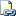# sp_sequence_get_range (Transact-SQL)

Returns a range of sequence values from a sequence object. The sequence object generates and issues the number of values requested and provides the application with metadata related to the range.Transact-SQL Syntax Conventions

## Syntax

``````sp_sequence_get_range [ @sequence_name = ] N'<sequence>'
, [ @range_size = ] range_size
, [ @range_first_value = ] range_first_value OUTPUT
[, [ @range_last_value = ] range_last_value OUTPUT ]
[, [ @range_cycle_count = ] range_cycle_count OUTPUT ]
[, [ @sequence_increment = ] sequence_increment OUTPUT ]
[, [ @sequence_min_value = ] sequence_min_value OUTPUT ]
[, [ @sequence_max_value = ] sequence_max_value OUTPUT ]
[ ; ]
``````

## Arguments

• [ @sequence_name = ] N'sequence'
The name of the sequence object. The schema is optional. sequence_name is nvarchar(776).

• [ @range_size = ] range_size
The number of values to fetch from the sequence. @range_size is bigint.

• [ @range_first_value = ] range_first_value
Output parameter returns the first (minimum or maximum) value of the sequence object used to calculate the requested range. @range_first_value is sql_variant with the same base type as that of the sequence object used in the request.

• [ @range_last_value = ] range_last_value
Optional output parameter returns the last value of the requested range. @range_last_value is sql_variant with the same base type as that of the sequence object used in the request.

• [ @range_cycle_count = ] range_cycle_count
Optional output parameter returns the number of times that the sequence object cycled in order to return the requested range. @range_cycle_count is int.

• [ @sequence_increment = ] sequence_increment
Optional output parameter returns the increment of the sequence object used to calculate the requested range. @sequence_increment is sql_variant with the same base type as that of the sequence object used in the request.

• [ @sequence_min_value = ] sequence_min_value
Optional output parameter returns the minimum value of the sequence object. @sequence_min_value is sql_variant with the same base type as that of the sequence object used in the request.

• [ @sequence_max_value = ] sequence_max_value
Optional output parameter returns the maximum value of the sequence object. @sequence_max_value is sql_variant with the same base type as that of the sequence object used in the request.

## Return Code Values

0 (success) or 1 (failure)

## Remarks

sp_sequence_get_range is in the sys. schema and can be referenced as sys.sp_sequence_get_range.

#### Cycling sequences

If required, the sequence object will cycle the appropriate number of times to service the requested range. The number of times cycled is returned to the caller through the @range\_cycle\_count parameter.

Note

When cycling, a sequence object restarts from the minimum value for an ascending sequence and the maximum value for a descending sequence, not from the start value of the sequence object.

#### Non-cycling sequences

If the number of values in the requested range is greater than the remaining available values in the sequence object the requested range is not deducted from the sequence object and the following error 11732 is returned:

The requested range for sequence object '%.*ls' exceeds the maximum or minimum limit. Retry with a smaller range.

## Permissions

Requires UPDATE permission on the sequence object or the schema of the sequence object.

## Examples

The following examples use a sequence object named Test.RangeSeq. Use the following statement to create the Test.RangeSeq sequence.

``````CREATE SCHEMA Test ;
GO

CREATE SEQUENCE Test.RangeSeq
AS int
INCREMENT BY 1
MINVALUE 1
MAXVALUE 25
CYCLE
CACHE 10
;
``````

### A. Retrieving a range of sequence values

The following statement gets four sequence numbers from the Test.RangeSeq sequence object and returns the first of the numbers to the user.

``````DECLARE @range_first_value sql_variant ,
@range_first_value_output sql_variant ;

EXEC sp_sequence_get_range
@sequence_name = N'Test.RangeSeq'
, @range_size = 4
, @range_first_value = @range_first_value_output OUTPUT ;

SELECT @range_first_value_output AS FirstNumber ;
``````

### B. Returning all output parameters

The following example returns all the output values from the sp_sequence_get_range procedure.

``````DECLARE
@FirstSeqNum sql_variant
, @LastSeqNum sql_variant
, @CycleCount int
, @SeqIncr sql_variant
, @SeqMinVal sql_variant
, @SeqMaxVal sql_variant ;

EXEC sys.sp_sequence_get_range
@sequence_name = N'Test.RangeSeq'
, @range_size = 5
, @range_first_value = @FirstSeqNum OUTPUT
, @range_last_value = @LastSeqNum OUTPUT
, @range_cycle_count = @CycleCount OUTPUT
, @sequence_increment = @SeqIncr OUTPUT
, @sequence_min_value = @SeqMinVal OUTPUT
, @sequence_max_value = @SeqMaxVal OUTPUT ;

-- The following statement returns the output values
SELECT
@FirstSeqNum AS FirstVal
, @LastSeqNum AS LastVal
, @CycleCount AS CycleCount
, @SeqIncr AS SeqIncrement
, @SeqMinVal AS MinSeq
, @SeqMaxVal AS MaxSeq ;
``````

Changing the @range\_size argument to a large number such as 75 will cause the sequence object to cycle. Check the @range\_cycle\_count argument to determine if and how many times the sequence object has cycled.

The following example gets a range from the Test.RangeSeq by using ADO.NET.

``````SqlCommand cmd = new SqlCommand();
cmd.Connection = conn;
cmd.CommandType = CommandType.StoredProcedure;
cmd.CommandText = "sys.sp_sequence_get_range";

// Specify an output parameter to retreive the first value of the generated range.
SqlParameter firstValueInRange = new SqlParameter("@range_first_value", SqlDbType.Variant);
firstValueInRange.Direction = ParameterDirection.Output;

conn.Open();
cmd.ExecuteNonQuery();

// Output the first value of the generated range.
Console.WriteLine(firstValueInRange.Value);
``````

#### Reference

CREATE SEQUENCE (Transact-SQL)

ALTER SEQUENCE (Transact-SQL)

DROP SEQUENCE (Transact-SQL)

NEXT VALUE FOR (Transact-SQL)

Sequence Numbers